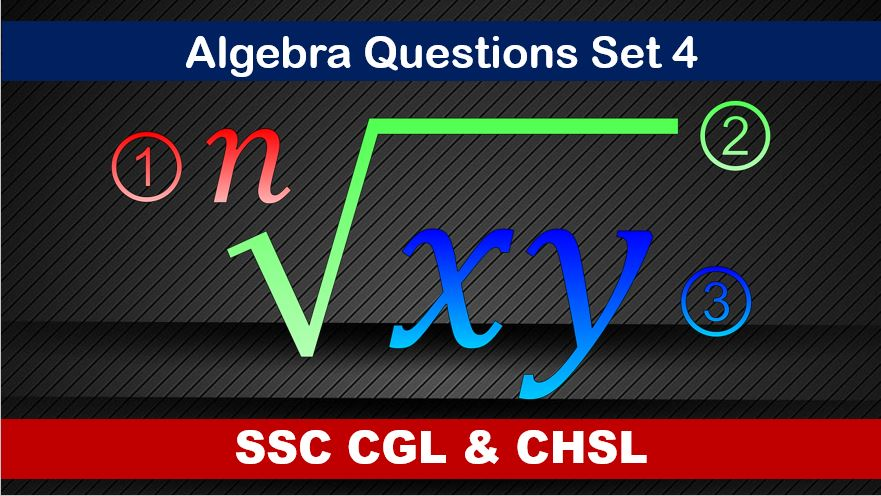Thursday, August 5, 2021
Home > algebra > SSC Algebra Questions and Solutions Set 4

# SSC Algebra Questions and Solutions Set 4SSC CGL CHSL Algebra
Algebra Questions Sets
Set 1Set 2Set 3Set 4Set 5
Set 6

SSC Algebra Questions and Solutions Set 4 : Ques No 1

If x – 1/x = 4, then the value of (x + 1/x) is

Options:

A. 5√2

B. 2√5

C. 4√2

D. 4√5

Solution:

SSC Algebra Questions and Solutions Set 4 : Ques No 2

If x = 3 + √8, then the value of (x2 + 1/x2) is

Options:

A. 34

B. 24

C. 38

D. 36

Solution:

SSC Algebra Questions and Solutions Set 4 : Ques No 3

If √(4x – 9) + √(4x + 9)  = 5 + √7, then the real value of x is

Options:

A. √5

B. 2√3

C. 3/√7

D. 4

Solution:

SSC Algebra Questions and Solutions Set 4 : Ques No 4

If the expression 9x2 – kx + 16 is perfect square, then the value of k is

Options:

A. ±12

B. ±24

C. ±2

D. ±6

Solution:

SSC Algebra Questions and Solutions Set 4 : Ques No 5

If a + b = 5, a2 + b2 = 13, then the value of (a – b), where a > b, is

Options:

A. 1

B. -1

C. 2

D. -2

Solution:

SSC Algebra Questions and Solutions Set 4 : Ques No 6

If a + 1/a = √3, then the value of a6 – 1/a6 + 2 is

Options:

A. 1

B. 2

C. 3√3

D. 5

Solution:

SSC Algebra Questions and Solutions Set 4 : Ques No 7

If x + y = √3 and x – y = √2, then the value of 8xy(x2 + y2) is

Options:

A. 5/9

B. 5

C. 5/2

D. √6

Solution:

SSC Algebra Questions and Solutions Set 4 : Ques No 8

If ax + by = 3, bx – ay = 4 and x2 + y2 = 1, then the value of a2 + b2 is

Options:

A. 17

B. 16

C. 9

D. 25

Solution:

SSC Algebra Questions and Solutions Set 4 : Ques No 9

If a2 + b2 + c2 = 2(a – b – c) – 3, then the value of 2a – 3b + 4c is

Options:

A. 3

B. 1

C. 2

D. 4

Solution:

SSC Algebra Questions and Solutions Set 4 : Ques No 10

If x = 2 + √3, y = 2 – √3, then the value of (x2 + y2) / (x3 + y3) is

Options:

A. 7/38

B. 7/40

C. 7/19

D. 7/26

Solution:

SSC Algebra Questions and Solutions Set 4 : Ques No 11

If x2 + y2 + 1/x2 + 1/y2 = 4, then the value of x2 + y2 is

Options:

A. 2

B. 7

C. 8

D. 16

SSC Algebra Questions and Solutions Set 4 : Ques No 12

If m + 1/(m-2) = 4, then the value of (m-2)2 + 1/(m-2)2 is

Options:

A. 1

B. 0

C. 2

D. 4

SSC Algebra Questions and Solutions Set 4 : Ques No 13

If x + y + z = 0, then the value of x2/yz + y2/zx + z2/xy is

Options:

A. (xyz)2

B. x2 + y2 + z2

C. 9

D. 3

SSC Algebra Questions and Solutions Set 4 : Ques No 14

If a + b + c = 0, then the value of (a2 + b2 + c2)/(a2 – bc) is

Options:

A. 0

B. 1

C. 2

D. 3

SSC Algebra Questions and Solutions Set 4 : Ques No 15

If x + 1/x = 99, then the value of 100x/(2x2 + 102x + 2)

Options:

A. 1/6

B. 1/2

C. 1/3

D. 1/4

SSC Algebra Questions and Solutions Set 4 : Ques No 16

If x + 1/2x = 99, then the value of 8×3 + 1/x3 is

Options:

A. 48

B. 80

C. 40

D. 44

SSC Algebra Questions and Solutions Set 4 : Ques No 17

If the average of x and 1/x is 1, then the value of 8x10 + 4/x5 is

Options:

A. 12

B. -12

C. 0

D. 1